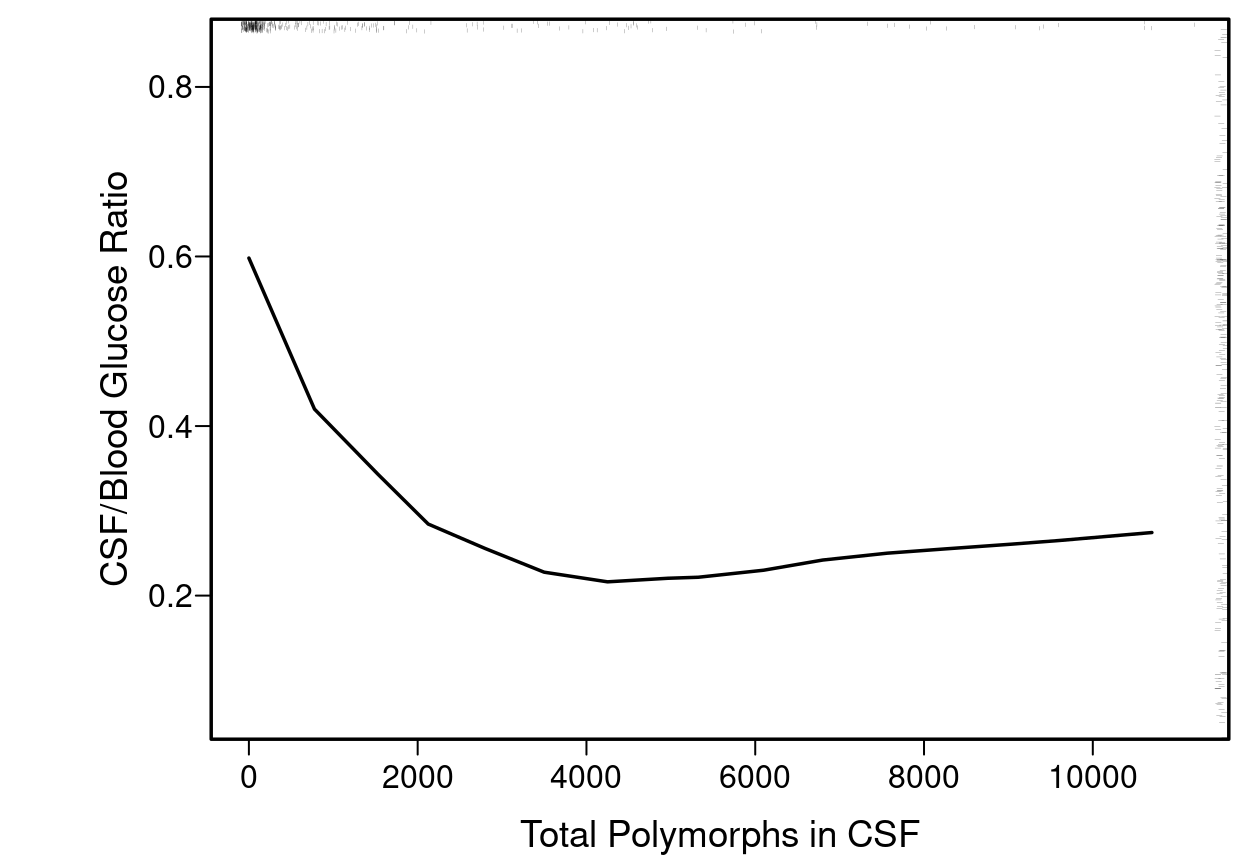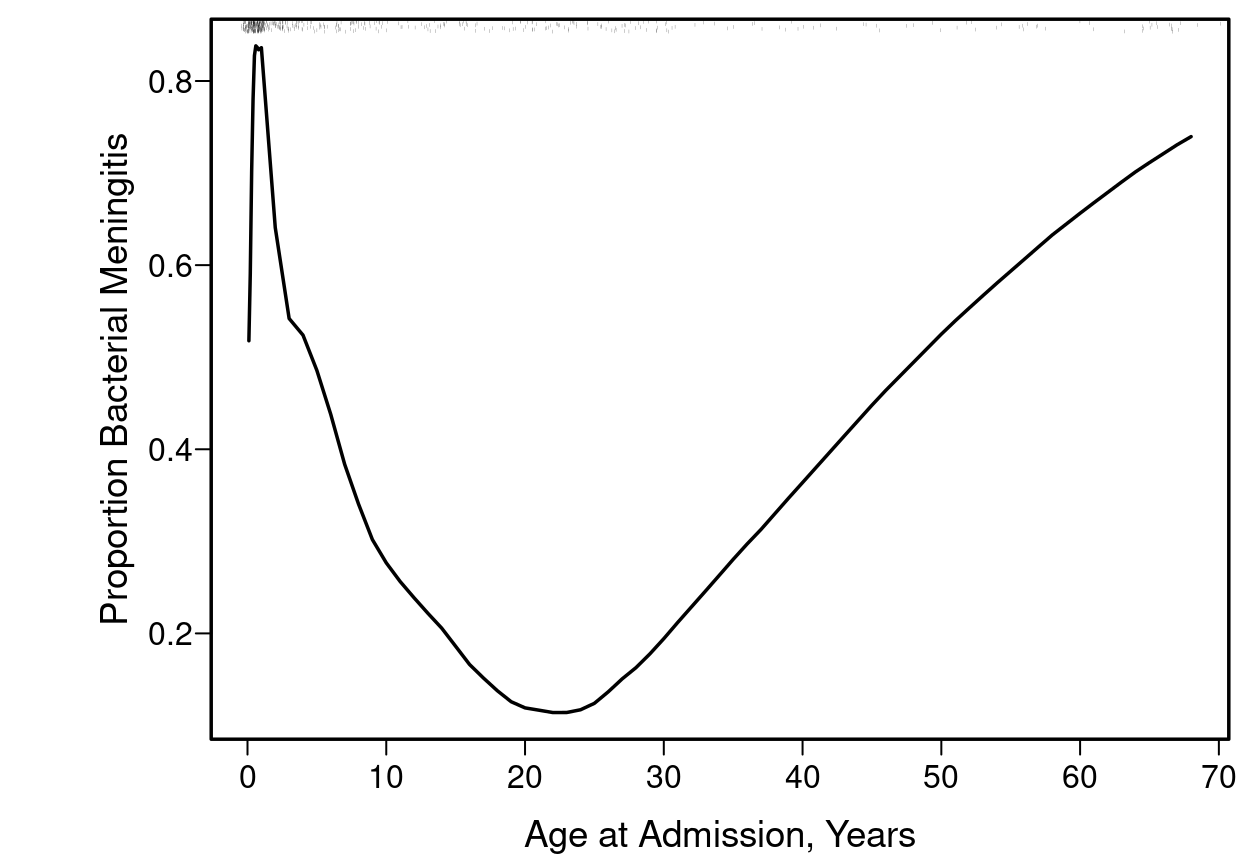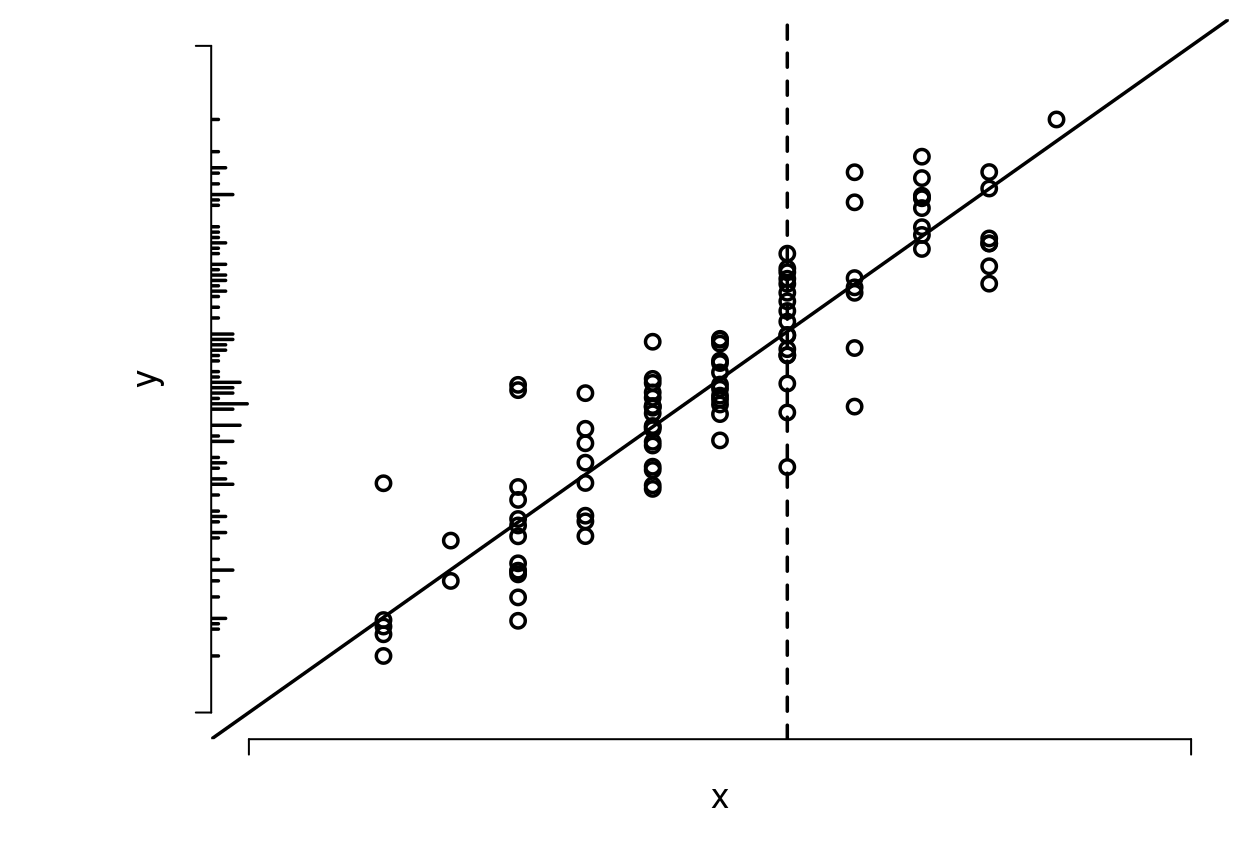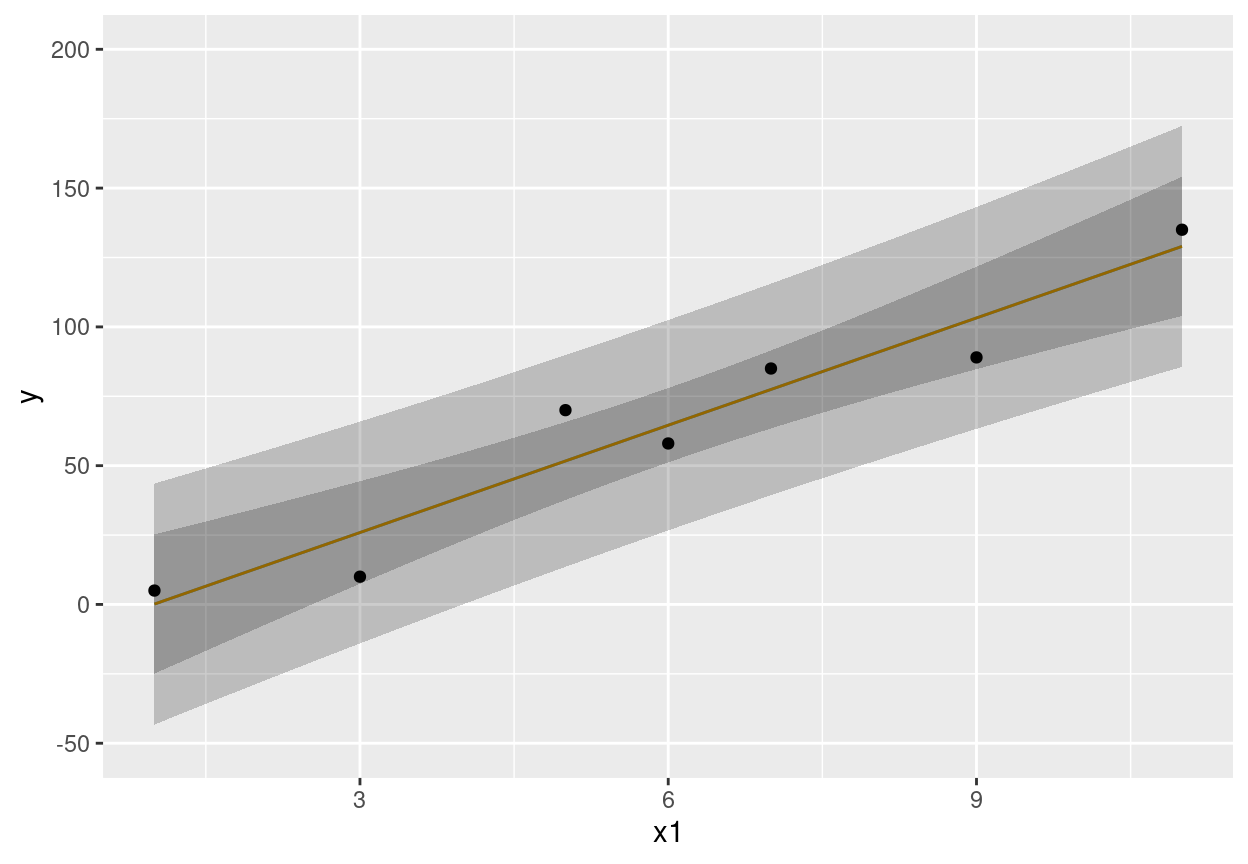# 10 Simple and Multiple Regression Models

Background

Regression models are used for

• hypothesis testing
• estimation
• prediction
• increasing power and precision for assessing the effect of one variable by adjusting for other variables that partially explain the outcome variable $$Y$$ (even in a randomized experiment with perfect covariate balance)
• confounder adjustment—getting adjusted estimates of effects
• checking that existing summary scores (e.g., BMI) adequately summarize their component variables
• fit a model with log height and log weight and see if ratio of coefficients is -2
• determining whether change, average, or most recent measurement should be emphasized
• fit a model containing body weight measured 1y ago and at time of treatment initiation
• if simple change score is an adequate summary of the two weights, the ratio of their coefficients will be about -1
• if most recent weight is all-important, coefficient for weight 1y ago will be very small
• developing new summary scores guided by predicting outcomes

Example

• Observational study of patients receiving treatments A and B
• Females are more likely to receive treatment B $$\rightarrow$$ need to adjust for sex
• Regression approach: fit a model with covariates (predictors) treatment and sex; treatment effect is adjusted for sex
• Stratification approach: for males estimate the B-A difference and do likewise for females
Average of the two differences is adjusted for sex

Now instead of sex being the relevant adjustment variable suppose it is age, and older patients tend to get treatment B

• Regression approach: fit a model with treatment and age
Treatment effect attempts to estimate the B-A difference at any chosen (fixed; conditioned on) age
• Stratification approach:
• divide age into quintiles
• within each quintile compute the B-A difference
• average these differences to get an almost age-adjusted treatment effect
• problem with residual heterogeneity of age within quintiles, especially at outer quintiles which are wider
• Matching approach:
• for each patient on A find a patient on B within 2y of same age
• if no match exists discard the A patient
• don’t use the same B patient again
• discard B patients who were not needed to match an A
• do a matched pairs analysis, e.g. paired $$t$$-test
• sample size is reduced $$\rightarrow \downarrow$$ power

## 10.1 Stratification vs. Matching vs. Regression

• Some ways to hold one variable $$x_1$$ constant when estimating the effect of another variable $$x_2$$ (covariable adjustment):
• experimental manipulation of $$x_1$$
• stratify the data on $$x_1$$ and for each stratum analyze the relationship between $$x_2$$ and $$Y$$
• form matched sets of observations on the basis of $$x_1$$ and use a statistical method applicable to matched data
• use a regression model to estimate the joint effects $$x_1$$ and $$x_2$$ have on $$Y$$; the estimate of the $$x_2$$ effect in the context of this model is essentially the $$x_2$$ relationship on $$Y'$$ where $$Y'$$ is $$Y$$ after the $$x_1$$ effect is subtracted from it
• Stratification and matching are not effective when $$x_1$$ is continuous as there are many $$x$$’s to hold constant
• Matching may be useful before data acquisition is complete or when sample is too small to allow for regression adjustment for $$>1$$ variable
• Matching after the study is completed usually results in discarding a large number of observations that would have been excellent matches
• Methods that discard information lose power and precision, and the observations discarded are arbitrary, damaging the study’s reproducibility
• Most matching methods depend on the row order of observations, again putting reproducibility into question
• There is no principled unique statistical approach to analysis of matched data
• All this points to many advantages of regression adjustment

Stratification and matching can be used to adjust for a small set of variables when assessing the association between a target variable and the outcome. Neither stratification nor matching are satisfactory when there are many adjustment variables or any of them are continuous. Crude stratification is often used in randomized trials to ensure that randomization stays balanced within subsets of subjects (e.g., males/females, clinical sites). Matching is an effective way to save resources before a study is done. For example, with a rare outcome one might sample all the cases and only twice as many controls as cases, matching controls to cases on age within a small tolerance such as 2 years. But once data are collected, matching is arbitrary, ineffective, and wasteful, and there are no principled unique statistical methods for analyzing matched data. For example if one wants to adjust for age via matching, consider these data:

Group Ages
Exposed 30 35 40 42
Unexposed 29 42 41 42

The matched sets may be constructed as follows:

Set Data
1 E30 U29
2 E40 U41
3 E42 U42a

U42a refers to the first 42 year old in the unexposed group. There is no match for E35. U42b was not used even though she was perfect match for E42.

1. Matching failed to interpolate for age 35; entire analysis must be declared as conditional on age not in the interval $$[36,39]$$
2. $$n \downarrow$$ by discarding observations that are easy to match (when the observations they are easily matched to were already matched)
3. Majority of matching algorithms are dependent on the row order of the dataset being analyzed so reproducibility is in question These problems combine to make post-sample-collection matching unsatisfactory from a scientific standpoint1.

Gelman has a nice chapter on matching.

RMS1.1

## 10.2 Purposes of Statistical Models

Statisticians, like artists, have the bad habit of falling in love with their models.
George E. P. Box
Most folk behave and thoughtlessly believe that the objective of analysis with statistical tools is to find / identify features in the data—period.

They assume and have too much faith that (1) the data effectively reflect nature and not noise, and (2) that statistical tools can ‘auto-magically’ divine causal relations.

They do not acknowledge that the objective of analysis should be to find interesting features inherent in nature (which to the extent that they indicate causal effects should be reproducible); represent these well; and use these features to make reliable decisions about future cases/situations.

Too often the p-value ‘satisfices’ for the intent of making reliable decisions about future cases/situations.

I also think that hypothesis testing and even point estimates for individual effects are really ersatz forms of prediction: ways of identifying and singling out a factor that allows people to make a prediction (and a decision) in a simple (simplistic) and expedient manner (again typically satisficing for cognitive ease). Well formulated prediction modeling is to be preferred to achieve this unacknowledged goal of making reliable decisions about future cases/situations on these grounds.
Drew Levy
@DrewLevy
March 2020

• Hypothesis testing
• Test for no association (correlation) of a predictor (independent variable) and a response or dependent variable (unadjusted test) or test for no association of predictor and response after adjusting for the effects of other predictors
• Estimation
• Estimate the shape and magnitude of the relationship between a single predictor (independent) variable and a response (dependent) variable
• Estimate the effect on the response variable of changing a predictor from one value to another
• Prediction
• Predicting response tendencies, e.g., long-term average response as a function of predictors
• Predicting responses of individual subjects

## 10.3 Advantages of Modeling

Even when only testing $$H_{0}$$ a model based approach has advantages:

• Permutation and rank tests not as useful for estimation
• Cannot readily be extended to cluster sampling or repeated measurements
• Models generalize tests
• 2-sample $$t$$-test, ANOVA →
multiple linear regression
• Wilcoxon, Kruskal-Wallis, Spearman →
proportional odds ordinal logistic model
• log-rank → Cox
• Models not only allow for multiplicity adjustment but for shrinkage of estimates
• Statisticians comfortable with $$P$$-value adjustment but fail to recognize that the difference between the most different treatments is badly biased Statistical estimation is usually model-based
• Relative effect of increasing cholesterol from 200 to 250 mg/dl on hazard of death, holding other risk factors constant
• Adjustment depends on how other risk factors relate to outcome
• Usually interested in adjusted (partial) effects, not unadjusted (marginal or crude) effects

## 10.4 Nonparametric Regression

RMS2.4.7

ABD17.8

• Estimate tendency (mean or median) of $$Y$$ as a function of $$X$$
• Few assumptions
• Especially handy when there is a single $$X$$
• Plotted trend line may be the final result of the analysis
• Simplest smoother: moving average
$$X$$: 1 2 3 5 8
$$Y$$: 2.1 3.8 5.7 11.1 17.2

$\begin{array}{ccc} \hat{E}(Y | X=2) &=& \frac{2.1+3.8+5.7}{3} \\ \hat{E}(Y | X=\frac{2+3+5}{3}) &=& \frac{3.8+5.7+11.1}{3} \end{array}$

• overlap OK
• problem in estimating $$E(Y)$$ at outer $$X$$-values
• estimates very sensitive to bin width
• Moving linear regression far superior to moving avg. (moving flat line)
• Cleveland’s moving linear regression smoother loess (locally weighted least squares) is the most popular smoother
• Example: differential diagnosis of acute bacterial meningitis vs. viral meningitis
require(Hmisc)
getHdata(abm)   # Loads data frame ABM (note case)
with(ABM, {
glratio <- gl / bloodgl
tpolys <- polys * whites / 100
plsmo(tpolys, glratio, xlab='Total Polymorphs in CSF',
ylab='CSF/Blood Glucose Ratio',
xlim=quantile(tpolys,  c(.05,.95), na.rm=TRUE),
ylim=quantile(glratio, c(.05,.95), na.rm=TRUE))
scat1d(tpolys); scat1d(glratio, side=4) })Figure 10.1: loess nonparametric smoother relating CSF:blood glucose ratio to total CSF polymorph count in patients with either bacterial or viral meningitis. Rug plot on axes plots indicate raw data values.

with(ABM, {
plsmo(age, abm, 'supsmu', bass=7,
xlab='Age at Admission, Years',
ylab='Proportion Bacterial Meningitis')
scat1d(age) })Figure 10.2: ‘Super smoother’’ relating age to the probability of bacterial meningitis given a patient has bacterial or viral meningitis, with a rug plot showing the age distribution.

## 10.5 Simple Linear Regression

A

ABD17-17.7,17.10-1

### 10.5.1 Notation

• $$y$$ : random variable representing response variable
• $$x$$ : random variable representing independent variable (subject descriptor, predictor, covariable)
• conditioned upon
• treating as constants, measured without error
• What does conditioning mean?
• holding constant
• subsetting on
• slicing scatterplot vertically
n <- 100
set.seed(13)
x <- round(rnorm(n, .5, .25), 1)
y <- x + rnorm(n, 0, .1)
r <- c(-.2, 1.2)
plot(x, y, axes=FALSE, xlim=r, ylim=r, xlab=expression(x), ylab=expression(y))
axis(1, at=r, labels=FALSE)
axis(2, at=r, labels=FALSE)
abline(a=0,b=1)
histSpike(y, side=2, add=TRUE)
abline(v=.6, lty=2)Figure 10.3: Data from a sample of $$n=100$$ points along with population linear regression line. The $$x$$ variable is discrete. The conditional distribution of $$y | x$$ can be thought of as a vertical slice at $$x$$. The unconditional distribution of $$y$$ is shown on the $$y$$-axis. To envision the conditional normal distributions assumed for the underlying population, think of a bell-shaped curve coming out of the page, with its base along one of the vertical lines of points. The equal variance assumption dictates that the series of Gaussian curves for all the different $$x$$s have equal variances.

• $$E(y|x)$$ : population expected value or long-run average of $$y$$ conditioned on the value of $$x$$
Example: population average blood pressure for a 30-year old

• $$\alpha$$ : $$y$$-intercept

• $$\beta$$ : slope of $$y$$ on $$x$$ ($$\frac{\Delta y}{\Delta x}$$) Simple linear regression is used when

B

• Only two variables are of interest

• One variable is a response and one a predictor

• No adjustment is needed for confounding or other between-subject variation

• The investigator is interested in assessing the strength of the relationship between $$x$$ and $$y$$ in real data units, or in predicting $$y$$ from $$x$$

• A linear relationship is assumed (why assume this? why not use nonparametric regression?)

• Not when one only needs to test for association (use Spearman’s $$\rho$$ rank correlation) or estimate a correlation index

### 10.5.2 Two Ways of Stating the Model

C

• $$E(y|x) = \alpha + \beta x$$
• $$y = \alpha + \beta x + e$$
$$e$$ is a random error (residual) representing variation between subjects in $$y$$ even if $$x$$ is constant, e.g. variation in blood pressure for patients of the same age

### 10.5.3 Assumptions, If Inference Needed

D
• Conditional on $$x$$, $$y$$ is normal with mean $$\alpha + \beta x$$ and constant variance $$\sigma^2$$, or:
• $$e$$ is normal with mean 0 and constant variance $$\sigma^2$$
• $$E(y|x) = E(\alpha + \beta x + e) = \alpha + \beta x + E(e)$$,
$$E(e) = 0$$.
• Observations are independent

### 10.5.4 How Can $$\alpha$$ and $$\beta$$ be Estimated?

E
• Need a criterion for what are good estimates
• One criterion is to choose values of the two parameters that minimize the sum of squared errors in predicting individual subject responses
• Let $$a, b$$ be guesses for $$\alpha, \beta$$
• Sample of size $$n: (x_{1}, y_{1}), (x_{2}, y_{2}), \ldots, (x_{n},y_{n})$$
• $$SSE = \sum_{i=1}^{n} (y_{i} - a - b x_{i})^2$$
• Values that minimize $$SSE$$ are least squares estimates
• These are obtained from

F
$\begin{array}{cc} L_{xx} = \sum(x_{i}-\bar{x})^2 & L_{xy} = \sum(x_{i}-\bar{x})(y_{i}-\bar{y}) \\ \hat{\beta} = b = \frac{L_{xy}}{L_{xx}} & \hat{\alpha} = a = \bar{y}- b \bar{x} \end{array}$

• Note: A term from $$L_{xy}$$ will be positive when $$x$$ and $$y$$ are concordant in terms of both being above their means or both being below their means.
• Least squares estimates are optimal if
1. the residuals truly come from a normal distribution
2. the residuals all have the same variance
3. the model is correctly specified, i.e., linearity holds
require(Hmisc)
getRs('demoLeastSquares.r')  # will load code into RStudio script editor
# click the Source button to run and follow
# instructions in console window
getRs('demoLeastSquares.r', put='source')   # if not using RStudio

### 10.5.5 Inference about Parameters

G

• Estimated residual: $$d = y - \hat{y}$$
• $$d$$ large if line was not the proper fit to the data or if there is large variability across subjects for the same $$x$$
• Beware of that many authors combine both components when using the terms goodness of fit and lack of fit
• Might be better to think of lack of fit as being due to a structural defect in the model (e.g., nonlinearity)
• $$SST = \sum_{i=1}^{n} (y_{i} - \bar{y}) ^ {2}$$
$$SSR = \sum (\hat{y}_{i} - \bar{y}) ^ {2}$$
$$SSE = \sum (y_{i} - \hat{y}_{i}) ^ 2$$
$$SST = SSR + SSE$$
$$SSR = SST - SSE$$
• $$SS$$ increases in proportion to $$n$$
• Mean squares: normalized for for d.f.: $$\frac{SS}{d.f.(SS)}$$
• $$MSR = SSR / p$$, $$p$$ = no. of parameters besides intercept (here, 1)
$$MSE = SSE / (n - p - 1)$$ (sample conditional variance of $$y$$)
$$MST = SST / (n - 1)$$ (sample unconditional variance of $$y$$)
• Brief review of ordinary ANOVA (analysis of variance):
• Generalizes 2-sample $$t$$-test to $$> 2$$ groups
• $$SSR$$ is $$SS$$ between treatment means
• $$SSE$$ is $$SS$$ within treatments, summed over treatments
• ANOVA Table for Regression
H
Source d.f. $$SS$$ $$MS$$ $$F$$
Regression $$p$$ $$SSR$$ $$MSR = SSR/p$$ $$MSR/MSE$$
Error $$n-p-1$$ $$SSE$$ $$MSE = SSE/(n-p-1)$$
Total $$n-1$$ $$SST$$ $$MST = SST/(n-1)$$
• Statistical evidence for large values of $$\beta$$ can be summarized by $$F = \frac{MSR}{MSE}$$
• Has $$F$$ distribution with $$p$$ and $$n-p-1$$ d.f.
• Large values $$\rightarrow |\beta|$$ large

### 10.5.6 Estimating $$\sigma$$, S.E. of $$\hat{\beta}$$; $$t$$-test

I
• $$s_{y\cdot x}^{2} = \hat{\sigma}^{2} = MSE = \widehat{Var}(y|x) = \widehat{Var}(e)$$
• $$\widehat{se}(b) = s_{y\cdot x}/L_{xx}^\frac{1}{2}$$
• $$t = b / \widehat{se}(b), n-p-1$$ d.f.
• $$t^{2} \equiv F$$ when $$p=1$$
• $$t_{n-2} \equiv \sqrt{F_{1, n-2}}$$
• $$t$$ identical to 2-sample $$t$$-test ($$x$$ has two values)
• If $$x$$ takes on only the values 0 and 1, $$b$$ equals ($$\bar{y}$$ when $$x=1$$) minus ($$\bar{y}$$ when $$x=0$$)

### 10.5.7 Interval Estimation

J

• 2-sided $$1-\alpha$$ CI for $$\beta$$: $$b \pm t_{n-2,1-\alpha/2} \widehat{se}(b)$$
• CI for predictions depend on what you want to predict even though $$\hat{y}$$ estimates both $$y$$2 and $$E(y|x)$$
• Notation for these two goals: $$\hat{y}$$ and $$\hat{E}(y|x)$$
• Predicting $$y$$ with $$\hat{y}$$ :
K

$$\widehat{s.e.}(\hat{y}) = s_{y \cdot x} \sqrt{1 + \frac{1}{n} + \frac{(x-\bar{x})^{2}}{L_{xx}}}$$
Note: This s.e. $$\rightarrow s_{y\cdot x}$$ as $$n \rightarrow \infty$$
• As $$n\rightarrow \infty$$, $$\widehat{s.e.}(\hat{y}) \rightarrow s_{y \cdot x}$$ which is $$> 0$$
• Fits with thinking of a predicted individual value being the predicted mean plus a randomly chosen residual (the former has SD going to zero as $$n \rightarrow \infty$$ and the latter has SD of $$s_{y \cdot x}$$)
• This is a valid predicted individual value but not the lowest mean squared error prediction which would be just to predict at the middle (the mean)
• Predicting $$\hat{E}(y|x)$$ with $$\hat{y}$$:
L

$$\widehat{s.e.}(\hat{E}(y|x)) = s_{y \cdot x} \sqrt{\frac{1}{n} + \frac{(x-\bar{x})^{2}}{L_{xx}}}$$ See footnote3
Note: This s.e. shrinks to 0 as $$n \rightarrow \infty$$
• $$1-\alpha$$ 2-sided CI for either one:
$$\hat{y} \pm t_{n-p-1,1-\alpha/2}\widehat{s.e.}$$
• Wide CI (large $$\widehat{s.e.}$$) due to:
• small $$n$$
• large $$\sigma^2$$
• being far from the data center ($$\bar{x}$$)
• Example usages:
• Is a child of age $$x$$ smaller than predicted for her age?
Use $$s.e.(\hat{y})$$
• What is the best estimate of the population mean blood pressure for patients on treatment $$A$$?
Use $$s.e.(\hat{E}(y|x))$$
• Example pointwise 0.95 confidence bands:
M

$$x$$ 1 3 5 6 7 9 11
$$y$$: 5 10 70 58 85 89 135
require(rms)
x1 <- c( 1,  3,  5,  6,  7,  9,  11)
y  <- c( 5, 10, 70, 58, 85, 89, 135)
dd <- datadist(x1, n.unique=5); options(datadist='dd')
f <- ols(y ~ x1)
p1 <- Predict(f, x1=seq(1,11,length=100), conf.type='mean')
p2 <- Predict(f, x1=seq(1,11,length=100), conf.type='individual')
p <- rbind(Mean=p1, Individual=p2)
ggplot(p, legend.position='none') +
geom_point(aes(x1, y), data=data.frame(x1, y, .set.=''))Figure 10.4: Pointwise 0.95 confidence intervals for $$\hat{y}$$ (wider bands) and $$\hat{E}(y|x)$$ (narrower bands).

### 10.5.8 Assessing Goodness of Fit

Assumptions:

1. Linearity
N
2. $$\sigma^2$$ is constant, independent of $$x$$
3. Observations ($$e$$’s) are independent of each other
4. For proper statistical inference (CI, $$P$$-values), $$e$$ is normal, or equivalently, $$y$$ is normal conditional on $$x$$

Verifying some of the assumptions:

O

• In a scattergram the spread of $$y$$ about the fitted line should be constant as $$x$$ increases, and $$y$$ vs. $$x$$ should appear linear
• Easier to see this with a plot of $$\hat{d} = y - \hat{y}$$ vs.
$$\hat{y}$$
• In this plot there are no systematic patterns (no trend in central tendency, no change in spread of points with $$x$$)
• Trend in central tendency indicates failure of linearity
• qqnorm plot of $$d$$
# Fit a model where x and y should have been log transformed
n <- 50
set.seed(2)
res <- rnorm(n, sd=.25)
x <- runif(n)
y <- exp(log(x) + res)
f <- ols(y ~ x)
plot(fitted(f), resid(f))
rl <- function() abline(h=0, col=gray(0.8))
rl()
# Fit a linear model that should have been quadratic
x <- runif(n, -1, 1)
y <- x ^ 2 + res
f <- ols(y ~ x)
plot(fitted(f), resid(f))
rl()
# Fit a correctly assumed linear model
y <- x + res
f <- ols(y ~ x)
plot(fitted(f), resid(f))
rl()
# Q-Q plot to check normality of residuals
qqnorm(resid(f)); qqline(resid(f))`